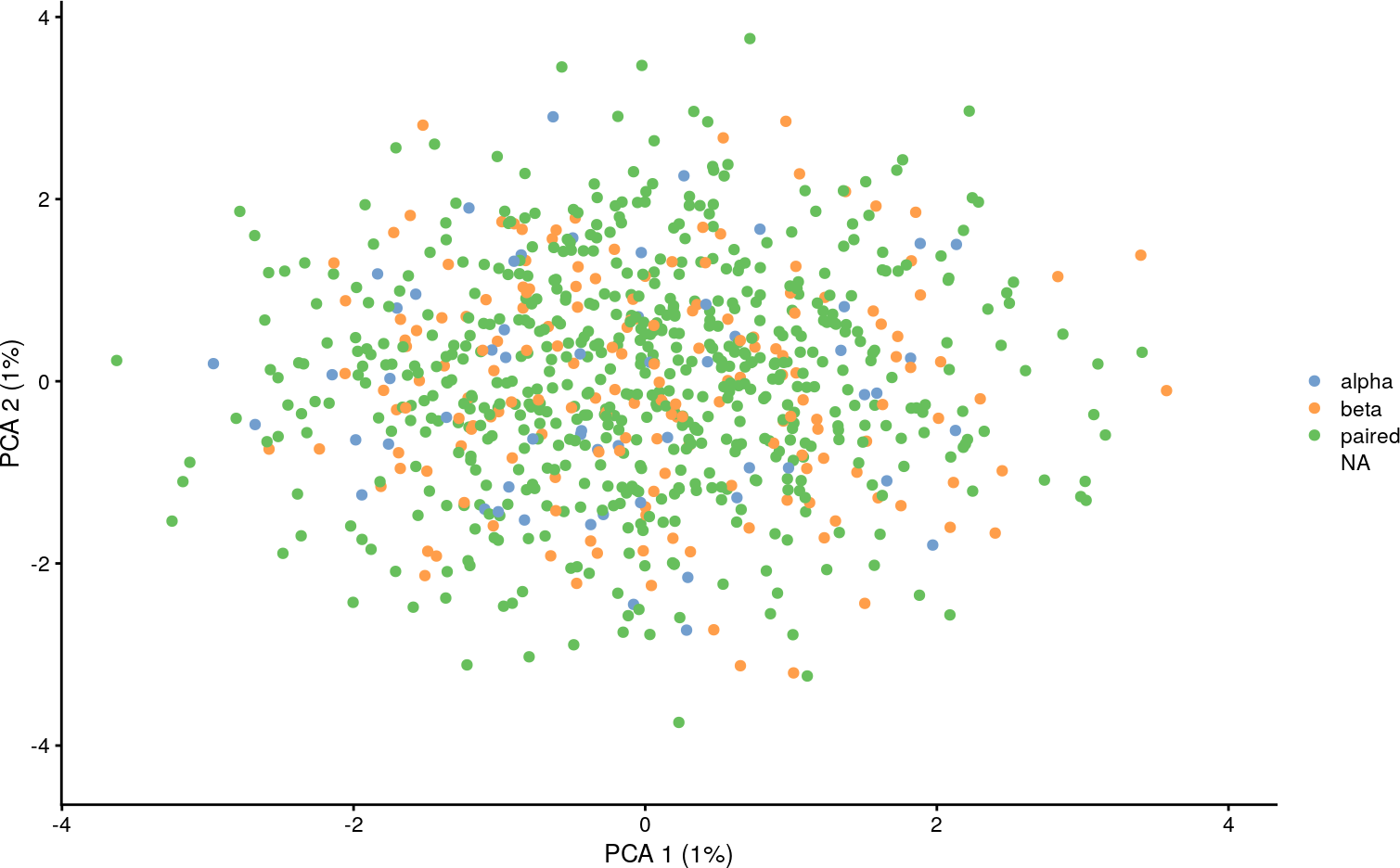# Contents

``````library(CellaRepertorium)
library(SingleCellExperiment)
#>
#> Attaching package: 'matrixStats'
#> The following object is masked from 'package:dplyr':
#>
#>     count
#>
#> Attaching package: 'MatrixGenerics'
#> The following objects are masked from 'package:matrixStats':
#>
#>     colAlls, colAnyNAs, colAnys, colAvgsPerRowSet, colCollapse,
#>     colCounts, colCummaxs, colCummins, colCumprods, colCumsums,
#>     colDiffs, colIQRDiffs, colIQRs, colLogSumExps, colMadDiffs,
#>     colMads, colMaxs, colMeans2, colMedians, colMins, colOrderStats,
#>     colProds, colQuantiles, colRanges, colRanks, colSdDiffs, colSds,
#>     colSums2, colTabulates, colVarDiffs, colVars, colWeightedMads,
#>     colWeightedMeans, colWeightedMedians, colWeightedSds,
#>     colWeightedVars, rowAlls, rowAnyNAs, rowAnys, rowAvgsPerColSet,
#>     rowCollapse, rowCounts, rowCummaxs, rowCummins, rowCumprods,
#>     rowCumsums, rowDiffs, rowIQRDiffs, rowIQRs, rowLogSumExps,
#>     rowOrderStats, rowProds, rowQuantiles, rowRanges, rowRanks,
#>     rowSdDiffs, rowSds, rowSums2, rowTabulates, rowVarDiffs, rowVars,
#>     rowWeightedSds, rowWeightedVars
#> Welcome to Bioconductor
#>
#>     Vignettes contain introductory material; view with
#>     'browseVignettes()'. To cite Bioconductor, see
#>     'citation("Biobase")', and for packages 'citation("pkgname")'.
#>
#> Attaching package: 'Biobase'
#> The following object is masked from 'package:MatrixGenerics':
#>
#>     rowMedians
#> The following objects are masked from 'package:matrixStats':
#>
#>     anyMissing, rowMedians
library(dplyr)
library(ggplot2)
library(tidyr)
library(stringr)
library(purrr)``````

It is possible to combine `ContigCellDB` objects with `SingleCellExperiment` objects that measure overlapping barcodes. We choose to include the `ContigCellDB` object as a member of the `colData`. In this way, it is possible to include different cellular “views” of the repertoire, such as the alpha chain and beta chain properties, as well as the paired clonotypes.

First we’ll cook up some single cell expression data.

``````set.seed(1345)
data(ccdb_ex)
barcodes = ccdb_ex\$cell_tbl[ccdb_ex\$cell_pk]

# Take a subsample of almost all of the barcdes
barcodes = barcodes[sample(nrow(barcodes), nrow(barcodes) - 5),]
samples = unique(ccdb_ex\$cell_tbl[setdiff(ccdb_ex\$cell_pk, 'barcode')])

# For each sample, generate  0-100 "extra" barcodes for which only 5' expression is recovered
extra = samples %>% rowwise() %>% mutate(extrabc = {
extra_bc = floor(runif(1, 0, 100))
list(tibble(barcode = paste0('barcode', seq_len(extra_bc))))
})
extra = extra %>% unnest(cols = c(extrabc))
all_bc = bind_rows(extra, barcodes)``````

Simulate some “cells” and “genes” that nearly form a superset of the cells for which repertoire are available. This is generally true if no barcode filters have been applied to the expression data. In practice a few cells may have repertoire but not expression (or fail QC for expression). We will work with the intersection of these cells.

``````genes = 200
cells = nrow(all_bc)
array_size = genes*cells
expression = matrix(rnbinom(array_size, size = 5, mu = 3), nrow = genes, ncol = cells)
sce = SingleCellExperiment(assay = list(counts = expression), colData = all_bc)``````

# 2 Remake the ContigCellDB with empty cells

``````ccdb2 = ccdb_join(sce, ccdb_ex)

ccdb2 = cdhit_ccdb(ccdb2, 'cdr3', type = 'AA', cluster_pk = 'aa80', identity = .8, min_length = 5)
ccdb2 = fine_clustering(ccdb2, sequence_key = 'cdr3', type = 'AA', keep_clustering_details = FALSE)
#> Calculating intradistances on 993 clusters.
#> Summarizing``````

The `ccdb_join(template, ccdb)` function does a left join of the `template` onto the `cell_tbl` of the `ccdb`. This will ensure that the `cell_tbl` is expanded and ordered properly to mesh with `sce` when we add it below.

# 3 Chain pairings

``````colData(sce)\$alpha =  canonicalize_cell(ccdb2, chain == 'TRA', contig_fields = c('chain', 'v_gene','d_gene', 'j_gene', 'aa80'))

colData(sce)\$beta =  canonicalize_cell(ccdb2, chain == 'TRB', contig_fields = c('chain', 'v_gene','d_gene', 'j_gene', 'aa80'))

colData(sce)\$pairing = enumerate_pairing(ccdb2, chain_recode_fun = 'guess')``````

We can add multiple views, represented as fields in the `colData(sce)` of the repertoire.

# 4 Visualization of TCR features with Scater

We can leverage Scater’s ability to use “nested” data frames to visualize TCR features.

``````library(scater)
sce = logNormCounts(sce)
sce = runPCA(sce)
plotReducedDim(sce, dimred = 'PCA', colour_by = I(sce\$pairing\$pairing), point_alpha = 1)
#> Warning: Removed 286 rows containing missing values (geom_point).``````Here we calculate the first two principal components (which aren’t very interesting because these are simulated data without any special structure), and then visualize if the TCR was paired or not.

``````only_paired = sce[,which(sce\$pairing\$pairing == 'paired')]
plotReducedDim(only_paired, dimred = 'PCA', colour_by = I(only_paired\$alpha\$j_gene), point_alpha = 1)
#> Warning: Removed 328 rows containing missing values (geom_point).``````